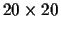Next: Deriving the Optimal Scoring Up: Dayhoff Scores and Evolutionary Previous: How to Make Scores

## How general are these scoring functions

A completely generic scoring function is

S(<a,b>) = G( Ea1b1, Ea2b2, ... )

where E is aarbitrary symmetric matrix and G is an arbitrary function which is symmetric in all its arguments. The symmetry of E is needed because when we inspect a mutation we cannot distinguish which mutated from which. Hence assigning different values to Eij and Eji is biologically incorrect. The symmetry of G is required because each mutation is unrelated to any other and does not depend on its position in the alignment. Therefore G depends only on the number of values Eij for each i and j. A brute force proof shows that for any symmetric polynomial of degree at most 3, the most efficient estimator is the same as for the linear case. At this time we are unable to prove that this holds for an arbitrary degree or for an arbitrary function, but we conjecture that the linear estimator (equation 6) is optimal for any symmetric function. Notice that it should be possible to approximate any reasonable estimator by an arbitrary degree polynomial, hence we conjecture that the estimator described here is optimal among all reasonable functions G.Next: Deriving the Optimal Scoring Up: Dayhoff Scores and Evolutionary Previous: How to Make Scores
Chantal Korostensky
1999-07-14